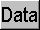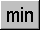calculator.org

# Statistical Functions

To access the statistical functions, make sure you are in Statistics mode; the title bar should end with [stats]. If not, click mode and select Statistics from the Number/Mode group. In statistics mode, the bottom right hand button on the calculator is data. To input values for analysis, enter numbers and click data after each number is input. Repeat this process until you have entered all the data values. If you press the shift button on the calculator, some of the number keys change to the statistics functions:For example, to get the number of points entered, press; to get the mean of the data, press thebutton.

To clear the statistics memory, press shift and then press SAC (Statistics All Clear) button (which was labelled AC before pressing shift).

##- Data Input

To input values for analysis, enter numbers and click data after each number is input. Repeat this process until you have entered all the data values.

##- Statistics All Clear

To clear the statistics memory, press the shift button, followed by SAC (i.e. shifted AC).

##- Permutations

Computes the number of permutations nPr.

Example:
To compute the number of ways of arranging five objects chosen from a population of ten:

1 0 shift5 =
Result: 30240

Note: The calculator must be in statistics mode.

##- Combinations

Computes the number of combinations nCr.

Example:
To compute the number of combinations of five objects chosen from a population of ten (without regard to the order of choosing):

1 0 shift5 =
Result: 252

Note: The calculator must be in statistics mode.

##- Maximum of data values

To obtain the maximum of all the values entered into the statistics memory, press shift then max.

##- Minimum of data values

To obtain the minimum of all the values entered into the statistics memory, press shift then min.

##- Number of Data Points

To obtain the total number of values entered into statistics memory, press shift then n.

##- Sum of Data

To obtain the sum of all the values entered into statistics memory, press shift then sum.

##- Sum of Squares of Data

To obtain the sum of the squares of all the values entered into statistics memory, press shift then ssq.

##- Average of Data

To obtain the mean (arithmetic average) of all the values entered into statistics memory, press shift then avg.

##- Data Standard Deviation

To obtain the standard deviation of all the values entered into statistics memory, press shift then sdv.

##- Data Sample Standard Deviation

To obtain the sample standard deviation of all the values entered into statistics memory, press shift then ssd.